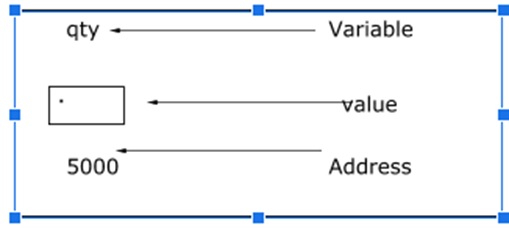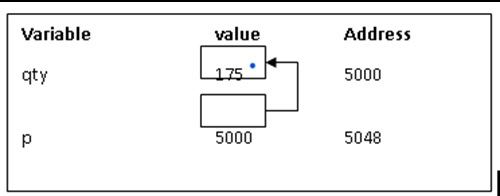# Explain the concept of pointer accessing in C language

Pointer is a variable which stores the address of other variable.

## Pointer declaration, initialization and accessing

Consider the following statement −

int qty = 179;## Declaring a pointer

int *p;

‘p’ is a pointer variable that holds the address of another integer variable.

## Initialization of a pointer

Address operator (&) is used to initialize a pointer variable.

int qty = 175;
int *p;
p= &qty;Let’s consider an example how the pointer is useful in accessing the elements in an array of string.

In this program, we are trying to access an element which is present at particular location. The location can be found by using an operation.

By adding pre incremented pointer to pre incremented pointer string and the subtracting 32, you get the value at that location.

## Example

Live Demo

#include<stdio.h>
int main(){
char s[] = {'a', 'b', 'c', '', 'c', '\0'};
char *p, *str, *str1;
p = &s;
str = p;
str1 = s;
printf("%d", ++*p + ++*str1-32);
return 0;
}

## Output

77

## Explanation

p = &s. i.e p = address of '';
str = p; i.e str = address of p;
str1 = s; str1 = address of 'a';
printf ("%d", ++*p + ++*str1 - 32);
i.e printf("%d", ++ + a -32);
i.e printf("%d", 12 + 97 -32);
i.e printf("%d", 12 + 65);
i.e printf("%d", 77);
Thus 77 is outputted